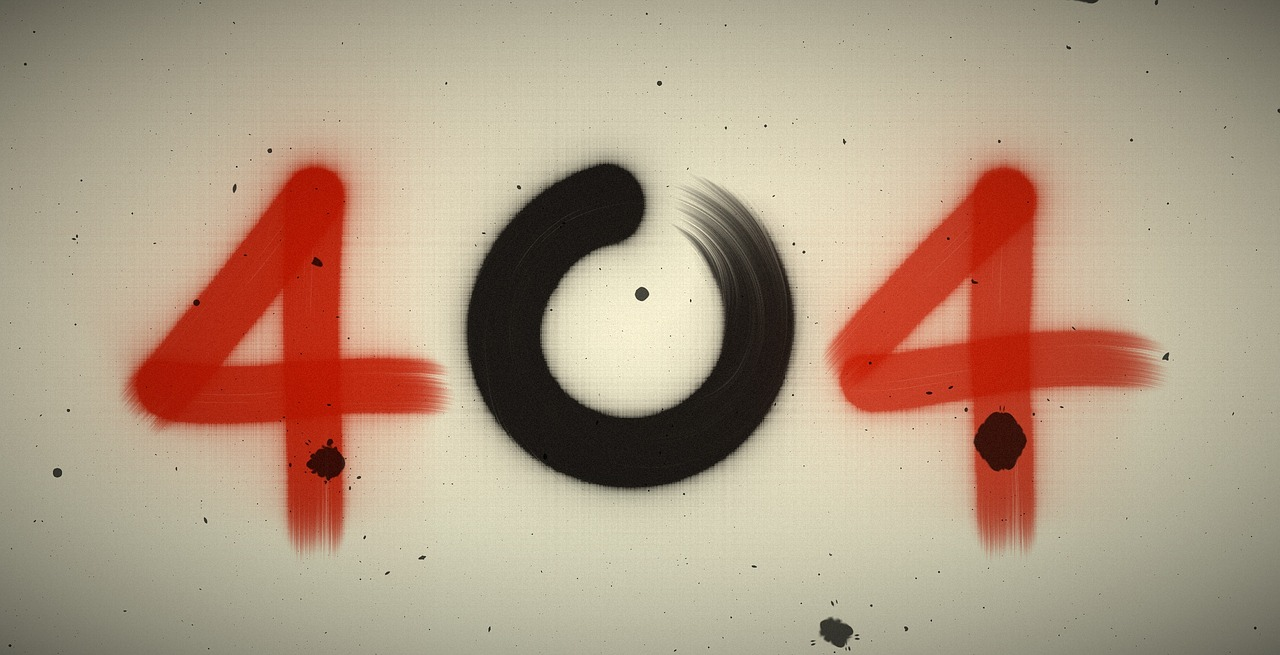## Post per Page

But why do certain elements have similar properties? Their properties have to do with the way electrons are arranged around the nucleus of atoms. But why are electrons arranged specifically in certain orbitals and shells? The structure of atoms can be predicted by quantum mechanics. It can explain the entire periodic table of elements.

Some configurations of electrons are more energy efficient than others. And this energy can be calculated using the Schrodinger equation. The lowest energy configurations of electrons is when the electron shells are either empty or full. What are atomic shells? The Bohr model of the hydrogen atom showed that electrons can only exist in certain stable orbits around a nucleus where its angular momentum is proportional to Planck’s constant. These are like shells around the nucleus.

Erwin Schrodinger showed that rather than being confined to an orbit like a planet, an electron is a matter wave that forms a probability cloud in 3D space smeared around the nucleus.

The Schrodinger equation showed that each shell has a maximum number of electrons it can hold. The inner most shell holds a maximum 2 electrons, the second 8 electrons, then 18, then 32, then 50, then 72 and so on. Chemistry is about substances exchanging or sharing electrons in order to fully fill up these shells

To understand why certain shells can only hold a specific number of electrons, we have to solve the Schrödinger equation, which is just a statement of energy conservation. It says that total energy is equal to potential energy plus kinetic energy. This equation can be solved most easily for the hydrogen atom because it is the simplest atom – just one electron orbiting one proton.

The key term to solve is psi, the wave function of the atom. It represents a value that is related to the probability of the atom being in certain quantum states. In order to solve these, you have to specify some quantum values for the atom, which are represented by n, l and m — n the electron shell layer, l is a quantum number defining the orbital angular momentum, and m specifies the orientation in space of the orbital.

When we plug in different values for n, l, and m in the Schrodinger equation for the hydrogen atom, it also approximately represents the solution to ALL the quantum states of electrons for any other atom. So, this equation allows us to predict how electrons behave for all the elements of the periodic table.

Different values of n, l and m can be shown to represent different structures of atoms because these quantum numbers explain the possible shells or orbitals available to the electrons.

We find that they total number of configurations is equal the electron shell numbers. So, the structure of the periodic table can be understood just by solving the Schrödinger equation for the hydrogen atom.

But can the hydrogen solution really work for all atoms? The answer no, this hydrogen solution isn’t exactly correct. It gets less accurate for larger atoms. So if you look in detail, there are some small variations for the larger atoms. And in those cases, the orbits can be occupied in a slightly different ways.

But the problem is that the Schrodinger equation becomes so unwieldy for large atoms, that it cannot be solved. But since we can solve it exactly for hydrogen, it can give us a good understanding for other atoms nevertheless.

Solving for the wave function also shows for example, the the orbits associated with a higher value of “l” are not necessarily the shells with the next lowest energy. This is why you learned in chemistry, that instead of starting the d-orbit in the third row, you start with the next s-orbit. The reason is because the wave function shows that when “n=4” and “l=0” in the s-orbit, it is actually more energy efficient than at the d-orbit. But after that, it becomes more efficient to fill up the d-orbitals.

So simply put, depending on the quantum numbers of the wavefunction, you fill up the orbits in terms of what requires the least energy. And voila, you get the periodic table! At the end of the day, chemistry is just quantum mechanics with electrons!